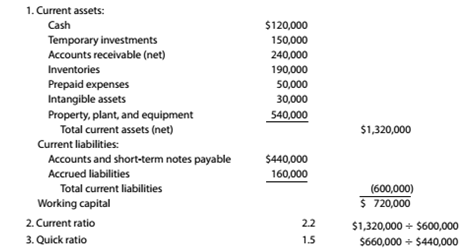Chapter 9, Problem 9.8E

Chapter
Section
Textbook Problem

Current position analysis The bond indenture for the 10-year. 8% debenture bonds dated January 2, 20Y8, required working capital of \$200,000. a current ratio of 2.0, and a quick ratio of 1.0 at the end of each calendar year until the bonds mature. At December 31. 20Y9. the three measures were computed as follows:a. List the errors in the determination of the three measures of current position analysis. b. Is the company satisfying the terms of the bond indenture?

To determine

Concept Introduction:

Working Capital: It is the difference between the current assets and the current liabilities of the business. Working capital is the amount which is used by the business to meet its short term expenses.

Current Ratio: Current ratio is the ratio of current assets with current liabilities it tells about the capacity of a company to meet its day to day expenses. Best current ratio is 2:1.

Quick Ratio: Quick ratio is the ratio of quick assets with current liabilities it tells about the liquidity of the company/ business. Best possible quick ratio is 1:1.

Requirement-1:

To Indicate:

List of three errors in the data.

Explanation

While calculating current assets we added intangible assets and plant, property and equipment which are non-current assets.

Correct Current assets = Cash+ Temporary investments+ Accounts receivables+ Inventories+ Prepaid Expenses...

To determine

Concept Introduction:

Working Capital: It is the difference between the current assets and the current liabilities of the business. Working capital is the amount which is used by the business to meet its short term expenses.

Current Ratio: Current ratio is the ratio of current assets with current liabilities it tells about the how much company strong to meet its day to day expenses. Best current ratio is 2:1.

Quick Ratio: Quick ratio is the ratio of quick assets with current liabilities it tells about the liquidity of the company/ business. Best possible quick ratio is 1:1.

Requirement-2:

To Indicate:

Is company satisfying the terms of the bond indenture

Still sussing out bartleby?

Check out a sample textbook solution.

See a sample solution

The Solution to Your Study Problems

Bartleby provides explanations to thousands of textbook problems written by our experts, many with advanced degrees!

Get Started

What are the five components of internal control?

College Accounting, Chapters 1-27 (New in Accounting from Heintz and Parry)

What is a value chain?

Pkg Acc Infor Systems MS VISIO CD

CONSTANT GROWTH Your broker offers to sell you some shares of Bahnsen Co. common stock that paid a dividend of...

Fundamentals of Financial Management, Concise Edition (with Thomson ONE - Business School Edition, 1 term (6 months) Printed Access Card) (MindTap Course List)